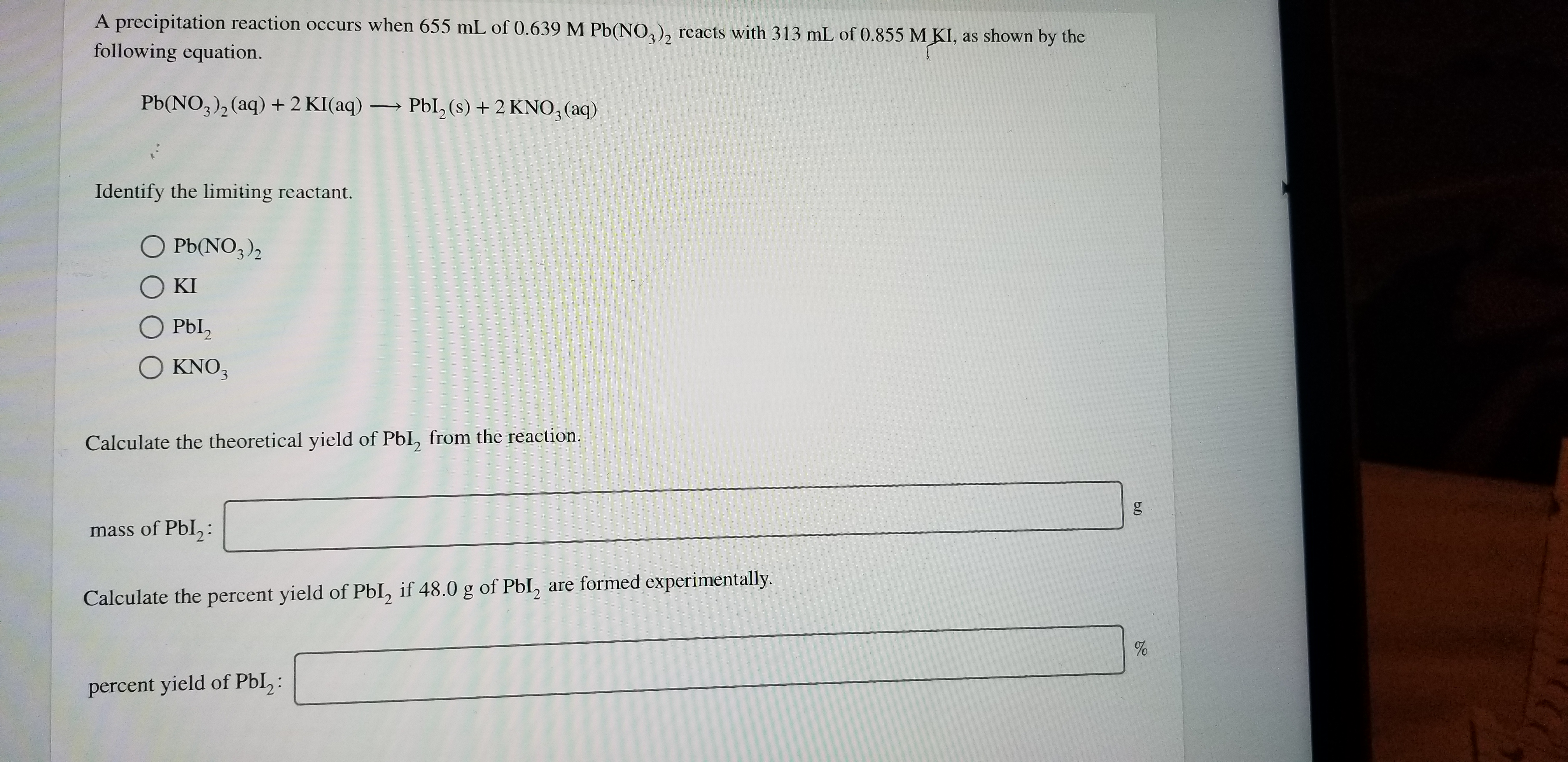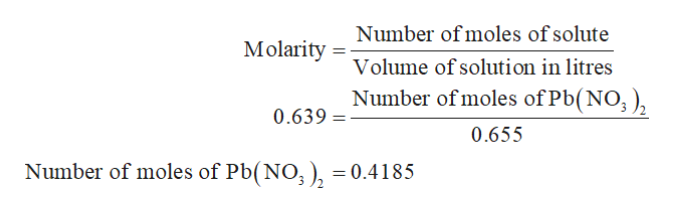# A precipitation reaction occurs when 655 mL of 0.639 M Pb(NO2), reacts with 313 mL of 0.855 M KI, as shown by thefollowing equation.Pb(NO3)2(aq) 2 KI(aq)Pbl, (s) + 2 KNO3 (aq)Identify the limiting reactant.O Pb(NO3)2O KIO Pbl2Ο ΚNO,KNO3Calculate the theoretical yield of PbI, from the reaction.mass of Pbl,:Calculate the percent yield of PbI, if 48.0 g of Pbl, are formed experimentally.percent yield of PbI,:

Question
236 viewshelp_outlineImage TranscriptioncloseA precipitation reaction occurs when 655 mL of 0.639 M Pb(NO2), reacts with 313 mL of 0.855 M KI, as shown by the following equation. Pb(NO3)2(aq) 2 KI(aq)Pbl, (s) + 2 KNO3 (aq) Identify the limiting reactant. O Pb(NO3)2 O KI O Pbl2 Ο ΚNO, KNO3 Calculate the theoretical yield of PbI, from the reaction. mass of Pbl,: Calculate the percent yield of PbI, if 48.0 g of Pbl, are formed experimentally. percent yield of PbI,: fullscreen
check_circle

Step 1

The given volume and molarity of Pb(NO3)2 is 655 mL and 0.639 M.

The given volume and molarity of KI is 313 mL and 0.855 M.

The given reaction is as follows:

Step 2

It is known that 1 mL = 0.001 L.

Therefore, the volume of Pb(NO3)2 in L is 0.655 L.

Similarly, the volume of KI in L is 0.313 L.

The mathematical expression of molarity is,

Step 3

Put the given values in the formula to calculate the nu...help_outlineImage TranscriptioncloseNumber of moles of solute Molarity Volume of solution in litres Number of moles of Pb(NO,) 0.639 0.655 Number of moles of Pb(NO3 ), = 0.4185 fullscreen

### Want to see the full answer?

See Solution

#### Want to see this answer and more?

Solutions are written by subject experts who are available 24/7. Questions are typically answered within 1 hour.*

See Solution
*Response times may vary by subject and question.
Tagged in

### Solutions